# 状态机（verilog）

---------------------------------------------------------------------------------------------------笔记（正点原子等）

Verilog是硬件描述语言，硬件电路是并行执行的，当需要按照流程或者步骤来完成某个

状态机，全称是有限状态机（Finite State Machine，缩写为FSM），是一种在有限个状

根据状态机的输出是否与输入条件相关，可将状态机分为两大类，即摩尔(Moore)型状态

➢ Mealy状态机：组合逻辑的输出不仅取决于当前状态，还取决于输入状态。

➢ Moore状态机：组合逻辑的输出只取决于当前状态。

（1）Mealy状态机

米勒状态机的模型如下图所示，模型中第一个方框是指产生下一状态的组合逻辑F，F是当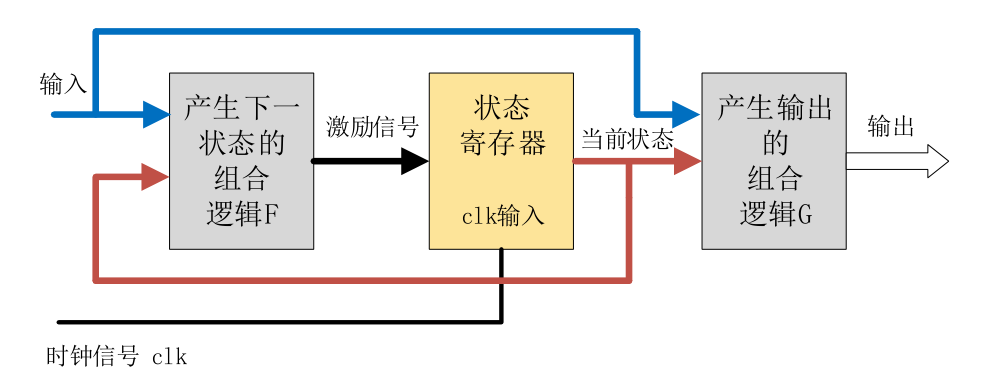（2）Moore状态机

摩尔状态机的模型如下图所示，对比米勒状态机的模型可以发现，其区别在于米勒状态机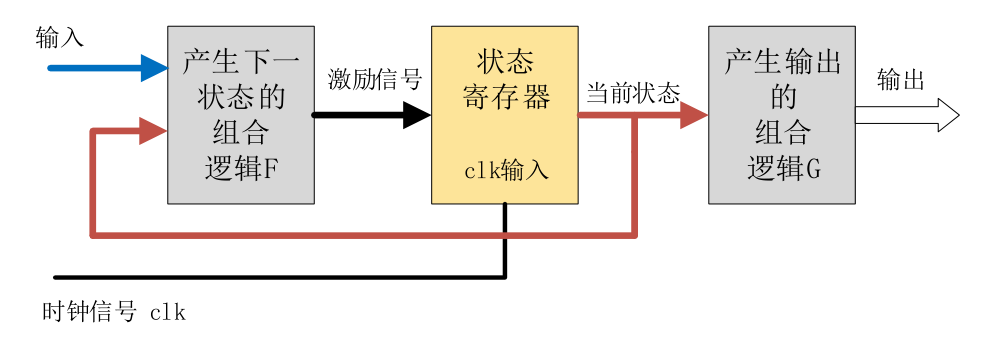三段式状态机：

根据状态机的实际写法，状态机还可以分为一段式、二段式和三段式状态机。
一段式：整个状态机写到一个always模块里面，在该模块中既描述状态转移又描述状态

。不推荐采用这种状态机，因为从代码风格方面来讲，一般都会要求把组合逻辑

二段式：用两个always模块来描述状态机，其中一个always模块采用同步时序描述状态转

三段式：在两个always模块描述方法基础上，使用三个always模块，一个always模块采用

实际应用中三段式状态机使用最多，因为三段式状态机将组合逻辑和时序分开，有利于综

；并且三段式状态机将状态转移与状态输出分开，使代码看上去

，推荐大家使用三段式状态机，本文也着重讲解三段式。

三段式状态机的基本格式是：
第一个always语句实现同步状态跳转；
第二个always语句采用组合逻辑判断状态转移条件；
第三个always语句描述状态输出(可以用组合电路输出，也可以时序电路输出)。

（1）七分频状态机：

在开始编写状态机代码之前，一般先画出状态跳转图，这样在编写代码时思路会比较清晰，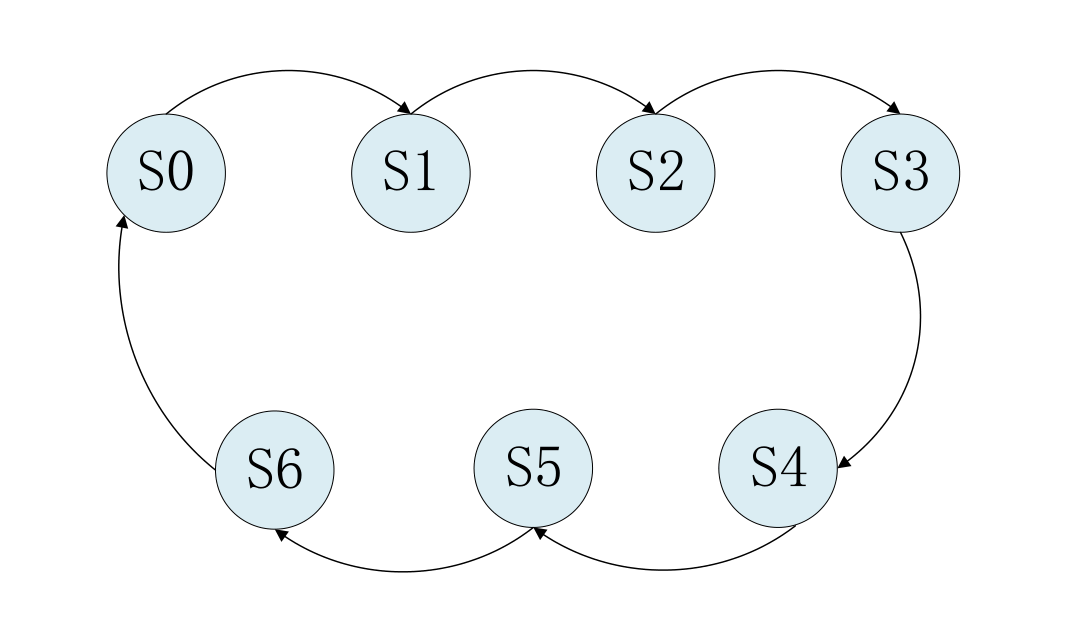状态跳转图画完之后，接下来通过parameter来定义各个不同状态的参数，如下代码所示：

parameter S0 = = 7'b0000001;  //独热码定义方式
parameter S1 = = 7'b0000010;
par ameter S2 = = 7'b0000100;
parameter S3 = = 7'b0001000;
parameter S4 = = 7'b0010000;
parameter S5 = = 7'b0100000;
parameter S6 = = 7'b1000000; 

这里是使用独热码的方式来定义状态机，每个状态只有一位为1，当然也可以直接定义成

因为我们定义成独热码的方式，每一个状态的位宽为7位，接下来还需要定义两个7位的寄

reg [ [6: :0] ] curr_st ; //当前状态
reg [ [6: :0] ] next_st ; //下一个状态

接下来就可以使用三个always语句来开始编写状态机的代码，第一个always采用同步时序

module divider7_fsm  (
//系统时钟与复位
input sys_clk ,
input sys_rst_n ,
//输出时钟
output reg clk_divide_7
);

//parameter define
parameter S0=7'b0000001; //独热码定义方式
parameter S1=7'b0000010;
parameter S2=7'b0000100;
parameter S3=7'b0001000;
parameter S4=7'b0010000;
parameter S5=7'b0100000;
parameter S6=7'b1000000;

//reg define
reg  [6:0]  curr_st;  //当前状态
reg  [6:0]  next_st; //下一个状态

//*****************************************************
//** main code
//*****************************************************

//状态机的第一段采用同步时序描述状态转移
always @( posedge sys_clk or negedge sys_rst_n ) begin
if (!sys_rst_n)
curr_st <= S0;
else
curr_st <= next_st;
end

//状态机的第二段采用组合逻辑判断状态转移条件
always @(*) begin
case ( (curr_st) )
S0:next_st = S1;
S1:next_st = S2;
S2:next_st = S3;
S3:next_st = S4;
S4:next_st = S5;
S5:next_st = S6;
S6:next_st = S0;
default: next_st = S0;
endcase
end

//状态机的第三段描述状态输出（这里采用时序电路输出）
always @( posedge sys_clk or negedge sys_rst_n) begin
if (!sys_rst_n)
clk_divide_7 <= 1'b0;
else if ((curr_st == S0)||( curr_st == S1 )||(curr_st == S2)||(curr_st == S3 ))
clk_divide_7 <= 1'b0;
else if ((curr_st == S4)||(curr_st == S5)||( curr_st == S6 ))
clk_divide_7 <= 1'b1;
else
clk_divide_7<=clk_divide_7;
end

endmodule

在编写状态机代码时首先要定义状态变量（代码中的参数S0~S6）与状态寄存器（curr_st、
next_st），如代码中第10行至第21行所示；接下来使用三个always语句来实现三段状态机，

从代码中可以看出，输出的分频时钟clk_divide_7只与当前状态（curr_st）有关，而与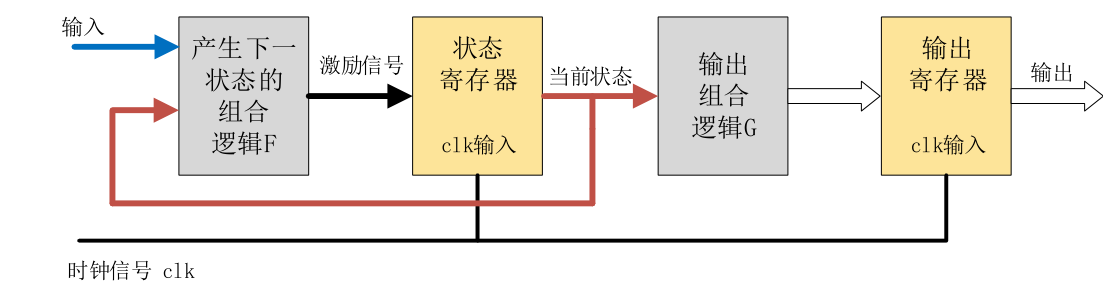采用这种描述方法虽然代码结构复杂了一些，但是这样做的好处是可以有效地滤去组合逻

（2）售卖机状态机：

◆自动售卖机的功能描述如下：

饮料单价 2 元，该售卖机只能接受 0.5 元、1 元的硬币。考虑找零和出货。投币和出货过程都是一次一次的进行，不会出现一次性投入多币或一次性出货多瓶饮料的现象。每一轮售卖机接受投币、出货、找零完成后，才能进入到新的自动售卖状态。

◆该售卖机的工作状态转移图如下所示，包含了输入、输出信号状态。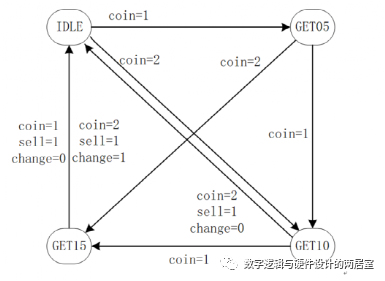◆状态机设计如下：

(0) 首先，根据状态机的个数确定状态机编码。利用编码给状态寄存器赋值，代码可读性更好。

(1) 状态机第一段，时序逻辑，非阻塞赋值，传递寄存器的状态。

(2) 状态机第二段，组合逻辑，阻塞赋值，根据当前状态和当前输入，确定下一个状态机的状态。

(3) 状态机第三代，时序逻辑，非阻塞赋值，因为是 Mealy 型状态机，根据当前状态和当前输入，确定输出信号。

// vending-machine
// 2 yuan for a bottle of drink
// only 2 coins supported: 5 jiao and 1 yuan
// finish the function of selling and changing

module  vending_machine_p3  (
input           clk ,
input           rstn ,
input [1:0]     coin ,     //01 for 0.5 jiao, 10 for 1 yuan

output [1:0]    change ,
output          sell    //output the drink
);

//machine state decode
parameter            IDLE   = 3'd0 ;
parameter            GET05  = 3'd1 ;
parameter            GET10  = 3'd2 ;
parameter            GET15  = 3'd3 ;

//machine variable
reg [2:0]            st_next ;
reg [2:0]            st_cur ;

//(1) state transfer
always @(posedge clk or negedge rstn) begin
if (!rstn) begin
st_cur      <= 'b0 ;
end
else begin
st_cur      <= st_next ;
end
end

//(2) state switch, using block assignment for combination-logic
//all case items need to be displayed completely
always @(*) begin
//st_next = st_cur ;//如果条件选项考虑不全，可以赋初值消除latch
case(st_cur)
IDLE:
case (coin)
2'b01:     st_next = GET05 ;
2'b10:     st_next = GET10 ;
default:   st_next = IDLE ;
endcase
GET05:
case (coin)
2'b01:     st_next = GET10 ;
2'b10:     st_next = GET15 ;
default:   st_next = GET05 ;
endcase

GET10:
case (coin)
2'b01:     st_next = GET15 ;
2'b10:     st_next = IDLE ;
default:   st_next = GET10 ;
endcase
GET15:
case (coin)
2'b01,2'b10:
st_next = IDLE ;
default:   st_next = GET15 ;
endcase
default:    st_next = IDLE ;
endcase
end

//(3) output logic, using non-block assignment
reg  [1:0]   change_r ;
reg          sell_r ;
always @(posedge clk or negedge rstn) begin
if (!rstn) begin
change_r       <= 2'b0 ;
sell_r         <= 1'b0 ;
end
else if ((st_cur == GET15 && coin ==2'h1)
|| (st_cur == GET10 && coin ==2'd2)) begin
change_r       <= 2'b0 ;
sell_r         <= 1'b1 ;
end
else if (st_cur == GET15 && coin == 2'h2) begin
change_r       <= 2'b1 ;
sell_r         <= 1'b1 ;
end
else begin
change_r       <= 2'b0 ;
sell_r         <= 1'b0 ;
end
end
assign       sell    = sell_r ;
assign       change  = change_r ;

endmodule

◆testbench 设计如下。

case1 对应连续输入 4 个 5 角硬币；

case2 对应 1 元 - 5 角 - 1 元的投币顺序；

case3 对应 5 角 - 1 元 - 5 角的投币顺序；

case4 对应连续 3 个 5 角然后一个 1 元的投币顺序。

timescale 1ns/1ps

module test ;
reg          clk;
reg          rstn ;
reg [1:0]    coin ;
wire [1:0]   change ;
wire         sell ;

//clock generating
parameter    CYCLE_200MHz = 10 ; //
always begin
clk = 0 ; #(CYCLE_200MHz/2) ;
clk = 1 ; #(CYCLE_200MHz/2) ;
end

//motivation generating
initial begin
coin      = 2'h0 ;
rstn      = 1'b0 ;
#8 rstn   = 1'b1 ;
@(negedge clk) ;

//case(1) 0.5 -> 0.5 -> 0.5 -> 0.5
#16 ;
repeat(5) begin
@(negedge clk) ;
end

//case(2) 1 -> 0.5 -> 1, taking change
#16 ;
repeat(5) begin
@(negedge clk) ;
end

//case(3) 0.5 -> 1 -> 0.5
#16 ;
repeat(5) begin
@(negedge clk) ;
end

//case(4) 0.5 -> 0.5 -> 0.5 -> 1, taking change
#16 ;
repeat(5) begin
@(negedge clk) ;
end
end

//(1) mealy state with 3-stage
vending_machine_p3    u_mealy_p3     (
.clk              (clk),
.rstn             (rstn),
.coin             (coin),
.change           (change),
.sell             (sell)
);

//simulation finish
always begin
#100;
if ($time >= 10000)$finish ;
end

endmodule 

◆仿真结果如下。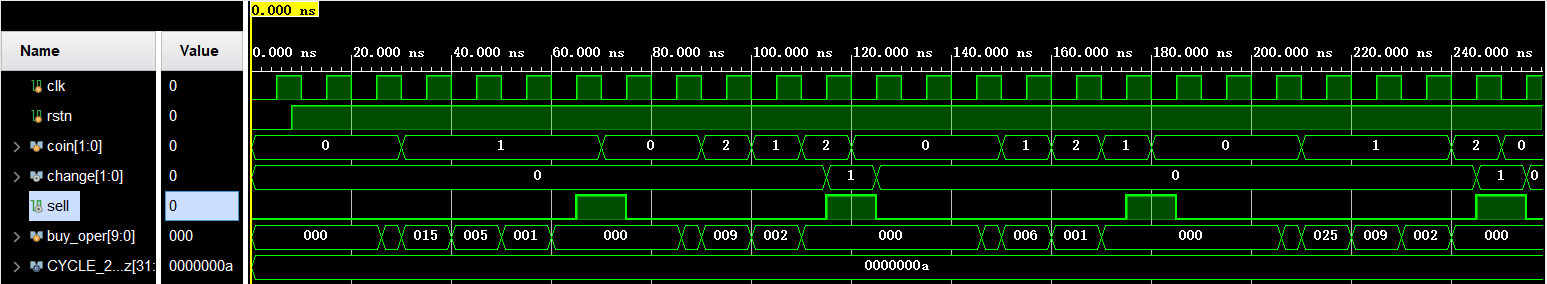◆将 3 段式状态机 2、3 段描述合并，其他部分保持不变，状态机就变成了 2 段式描述。

 //(2) state switch, and output logic
//all using block assignment for combination-logic
reg  [1:0]   change_r ;
reg          sell_r ;
always @(*) begin //all case items need to be displayed completely
case(st_cur)
IDLE: begin
change_r     = 2'b0 ;
sell_r       = 1'b0 ;
case (coin)
2'b01:     st_next = GET05 ;
2'b10:     st_next = GET10 ;
default:   st_next = IDLE ;
endcase // case (coin)
end
GET05: begin
change_r     = 2'b0 ;
sell_r       = 1'b0 ;
case (coin)
2'b01:     st_next = GET10 ;
2'b10:     st_next = GET15 ;
default:   st_next = GET05 ;
endcase // case (coin)
end

GET10:
case (coin)
2'b01:     begin
st_next      = GET15 ;
change_r     = 2'b0 ;
sell_r       = 1'b0 ;
end
2'b10:     begin
st_next      = IDLE ;
change_r     = 2'b0 ;
sell_r       = 1'b1 ;
end
default:   begin
st_next      = GET10 ;
change_r     = 2'b0 ;
sell_r       = 1'b0 ;
end
endcase // case (coin)

GET15:
case (coin)
2'b01: begin
st_next     = IDLE ;
change_r    = 2'b0 ;
sell_r      = 1'b1 ;
end
2'b10:     begin
st_next     = IDLE ;
change_r    = 2'b1 ;
sell_r      = 1'b1 ;
end
default:   begin
st_next     = GET15 ;
change_r    = 2'b0 ;
sell_r      = 1'b0 ;
end
endcase
default:  begin
st_next     = IDLE ;
change_r    = 2'b0 ;
sell_r      = 1'b0 ;
end

endcase
end

◆将上述修改的新模块例化到 3 段式的 testbench 中即可进行仿真，结果如下。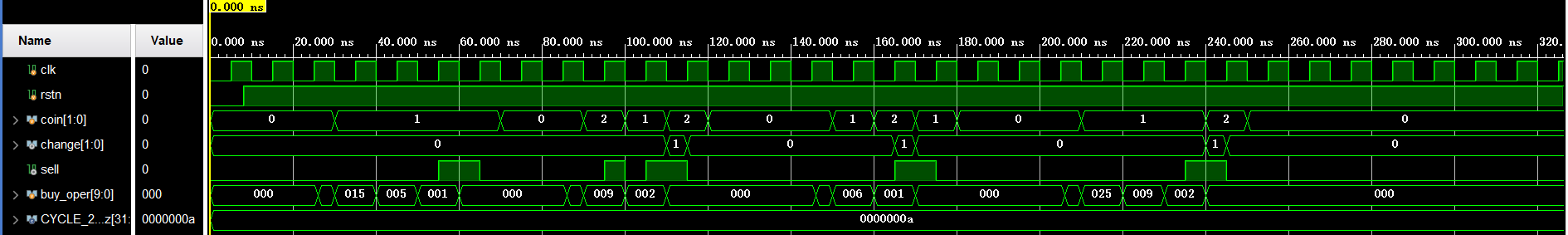◆将 3 段式状态机 1、 2、3 段描述合并，状态机就变成了 1 段式描述。

//(1) using one state-variable do describe
reg  [1:0]   change_r ;
reg          sell_r ;
always @(posedge clk or negedge rstn) begin
if (!rstn) begin
st_cur     <= 'b0 ;
change_r   <= 2'b0 ;
sell_r     <= 1'b0 ;
end
else begin
case(st_cur)

IDLE: begin
change_r  <= 2'b0 ;
sell_r    <= 1'b0 ;
case (coin)
2'b01:     st_cur <= GET05 ;
2'b10:     st_cur <= GET10 ;
endcase
end
GET05: begin
case (coin)
2'b01:     st_cur <= GET10 ;
2'b10:     st_cur <= GET15 ;
endcase
end

GET10:
case (coin)
2'b01:     st_cur   <=  GET15 ;
2'b10:     begin
st_cur   <= IDLE ;
sell_r   <= 1'b1 ;
end
endcase

GET15:
case (coin)
2'b01:     begin
st_cur   <= IDLE ;
sell_r   <= 1'b1 ;
end
2'b10:     begin
st_cur   <= IDLE ;
change_r <= 2'b1 ;
sell_r   <= 1'b1 ;
end
endcase

default:  begin
st_cur    <= IDLE ;
end

endcase // case (st_cur)
end // else: !if(!rstn)
end

◆将上述修改的新模块例化到 3 段式的 testbench 中即可进行仿真，结果如下。

1 段式状态机的缺点就是许多种逻辑糅合在一起，不易后期的维护。当状态机和输出信号较少时，可以尝试此种描述方式。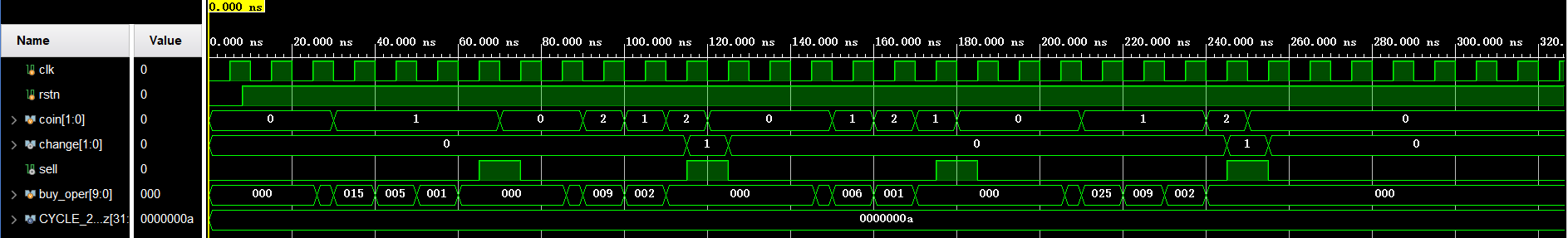3 段式 Moore 型状态机描述的自动售卖机 Verilog 代码如下：

module  vending_machine_moore    (
input           clk ,
input           rstn ,
input [1:0]     coin ,     //01 for 0.5 jiao, 10 for 1 yuan
output [1:0]    change ,
output          sell    //output the drink
);

//machine state decode
parameter            IDLE   = 3'd0 ;
parameter            GET05  = 3'd1 ;
parameter            GET10  = 3'd2 ;
parameter            GET15  = 3'd3 ;
// new state for moore state-machine
parameter            GET20  = 3'd4 ;
parameter            GET25  = 3'd5 ;

//machine variable
reg [2:0]            st_next ;
reg [2:0]            st_cur ;

//(1) state transfer
always @(posedge clk or negedge rstn) begin
if (!rstn) begin
st_cur      <= 'b0 ;
end
else begin
st_cur      <= st_next ;
end
end

//(2) state switch, using block assignment for combination-logic
always @(*) begin //all case items need to be displayed completely
case(st_cur)
IDLE:
case (coin)
2'b01:     st_next = GET05 ;
2'b10:     st_next = GET10 ;
default:   st_next = IDLE ;
endcase
GET05:
case (coin)
2'b01:     st_next = GET10 ;
2'b10:     st_next = GET15 ;
default:   st_next = GET05 ;
endcase

GET10:
case (coin)
2'b01:     st_next = GET15 ;
2'b10:     st_next = GET20 ;
default:   st_next = GET10 ;
endcase
GET15:
case (coin)
2'b01:     st_next = GET20 ;
2'b10:     st_next = GET25 ;
default:   st_next = GET15 ;
endcase
GET20:         st_next = IDLE ;
GET25:         st_next = IDLE ;
default:       st_next = IDLE ;
endcase // case (st_cur)
end // always @ (*)

// (3) output logic,
// one cycle delayed when using non-block assignment
reg  [1:0]   change_r ;
reg          sell_r ;
always @(posedge clk or negedge rstn) begin
if (!rstn) begin
change_r       <= 2'b0 ;
sell_r         <= 1'b0 ;
end
else if (st_cur == GET20 ) begin
sell_r         <= 1'b1 ;
end
else if (st_cur == GET25) begin
change_r       <= 2'b1 ;
sell_r         <= 1'b1 ;
end
else begin
change_r       <= 2'b0 ;
sell_r         <= 1'b0 ;
end
end
assign       sell    = sell_r ;
assign       change  = change_r ;

endmodule


◆将上述修改的 Moore 状态机例化到 3 段式的 testbench 中即可进行仿真，结果如下。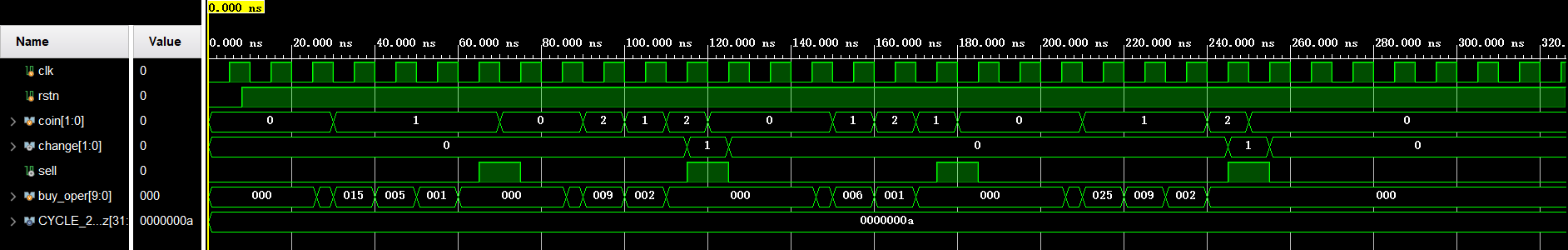◆输出信号赋值时，用阻塞赋值，则可以提前一个时钟周期。

// (3.2) output logic, using block assignment
reg  [1:0]   change_r ;
reg          sell_r ;
always @(*) begin
change_r  = 'b0 ;
sell_r    = 'b0 ; //not list all condition, initializing them
if (st_cur == GET20 ) begin
sell_r         = 1'b1 ;
end
else if (st_cur == GET25) begin
change_r       = 2'b1 ;
sell_r         = 1'b1 ;
end
end`

◆输出信号阻塞赋值的仿真结果如下。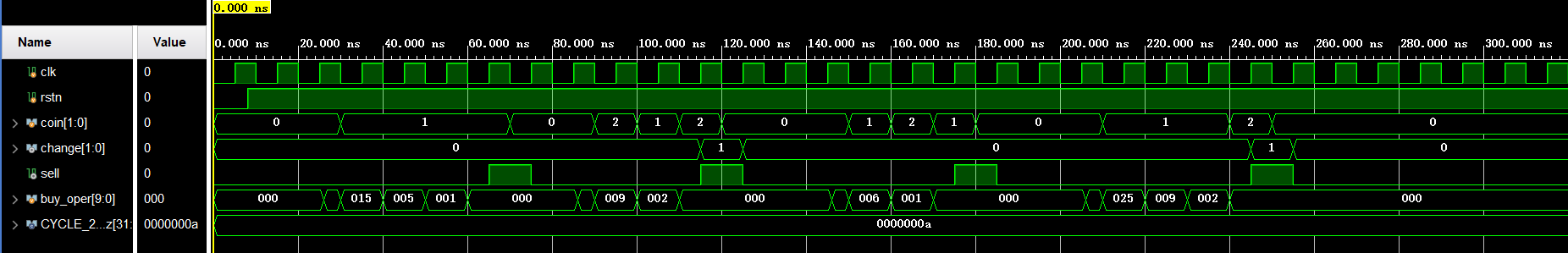09-191060
08-119万+

09-127123
02-041466
04-252201
10-134201
09-291810
07-27632
08-301539
07-143万+
10-231500
10-201015
04-251166
08-211282
05-16290
02-173597
06-175792
09-302236
05-10109
04-034078

### “相关推荐”对你有帮助么？

•非常没帮助
•没帮助
•一般
•有帮助
•非常有帮助¥2 ¥4 ¥6 ¥10 ¥20余额支付 (余额：-- )扫码支付获取中扫码支付点击重新获取扫码支付1.余额是钱包充值的虚拟货币，按照1:1的比例进行支付金额的抵扣。
2.余额无法直接购买下载，可以购买VIP、C币套餐、付费专栏及课程。余额充值